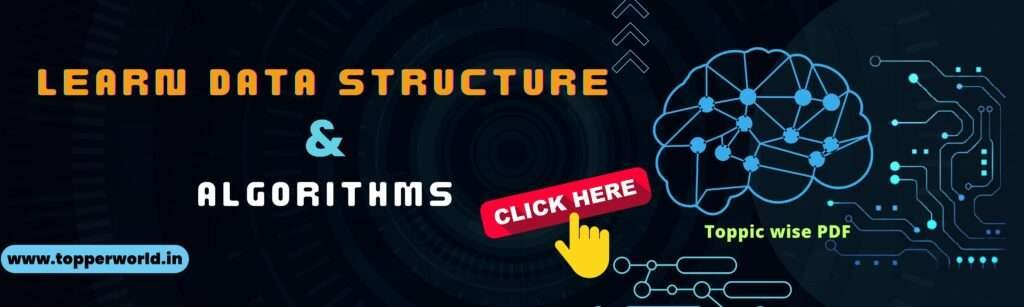### Graph Data Structure

Graphs are a fundamental data structure used in computer science and various fields to represent and analyze relationships between objects. Whether modeling social networks, transportation systems, or optimizing routes in GPS applications, graphs play a crucial role. In this, we’ll explore the concept of a graph data structure, understand its components, and delve into its practical applications.

A graph is a collection of nodes (vertices) and edges that connect these nodes. Each edge represents a relationship or connection between two nodes. Graphs can be used to model a wide range of scenarios where relationships or connections are essential.

In the below PDF we discuss about Graph Data Structure in detail in simple language, Hope this will help in better understanding.

Topics Covered inside the PDF :

• Graph Implementation
• BFS Algorithm
• DFS Algorithm
• Spanning Tree#### Components of a Graph :

To understand graphs better, let’s break down their key components:

• Vertices (Nodes): Vertices are the fundamental units of a graph. They represent entities or objects in the dataset. In a social network graph, each user may be represented as a vertex.
• Edges: Edges are the connections between vertices. They define the relationships or interactions between the entities. For example, in a transportation network graph, edges could represent roads or flight paths.
• Weight: Some graphs have weighted edges, where each edge is assigned a numerical value, indicating a cost, distance, or any relevant metric. Weighted graphs are commonly used in optimization problems.
• Directed vs. Undirected: Graphs can be classified as directed or undirected. In a directed graph, edges have a direction, going from one vertex to another. In an undirected graph, edges have no direction and simply represent a connection between two vertices.
• Cycles: A cycle in a graph is a path that starts and ends at the same vertex, traversing a sequence of edges. Detecting cycles is crucial in many applications, such as deadlock detection in computer networks.

#### Types of Graphs

Graphs can take various forms, depending on their characteristics:

• Simple Graph: A graph with no self-loops (edges connecting a vertex to itself) and no multiple edges between the same pair of vertices.
• Directed Graph (Digraph): A graph in which edges have a direction, going from one vertex to another.
• Weighted Graph: A graph with edges that have numerical weights or values associated with them.
• Connected Graph: A graph in which there is a path between every pair of vertices.
• Tree: A connected, acyclic (no cycles) graph with one designated vertex as the root. Trees are used in hierarchical structures like family trees and file systems.

#### Practical Applications :

Graph data structures find applications in various domains:

• Social Networks: Social media platforms use graphs to model connections between users and recommend friends or content.
• Transportation: Graphs are employed to model road networks, flight routes, and public transportation systems for route planning and optimization.
• Recommendation Systems: E-commerce and content streaming services use graphs to recommend products, movies, or music based on user preferences and connections.
• Networks and Telecommunications: Graphs represent data flow, connections, and routing in computer networks and telecommunications systems.
• Bioinformatics: Graphs are used to model molecular structures, protein-protein interactions, and genetic relationships.
• Semantic Web: In the Semantic Web, graphs represent linked data and ontologies, enabling meaningful relationships between information.
• Cybersecurity: Graphs help in detecting network anomalies, identifying attack patterns, and visualizing network traffic.

#### Related Question

A graph data structure is a collection of nodes (vertices) and edges that connect these nodes. It is used to represent and analyze relationships or connections between objects.

Vertices (or nodes) are the fundamental units of a graph, representing entities or objects. Edges are the connections between vertices, indicating relationships or interactions.

In a directed graph, edges have a direction, going from one vertex to another. In an undirected graph, edges have no direction and simply represent a connection between two vertices.

Weighted edges in a graph assign numerical values to edges, indicating a cost, distance, or any relevant metric. Weighted graphs are used in optimization problems where finding the best path or solution is essential.

A tree is a type of graph that is connected and acyclic, meaning it has no cycles (loops). Trees have one designated vertex as the root, and they are commonly used in hierarchical structures like family trees and file systems.

Graph data structures find applications in various domains, including social networks, transportation systems, recommendation systems, bioinformatics, semantic web, cybersecurity, and more.

#### DSA interview Questions

DSA Inetrview Questions Are you

#### Recursion in Data Structure

Recursion in Data Structure Recursion

#### Tree Data Structure

Tree Data Structure Trees are